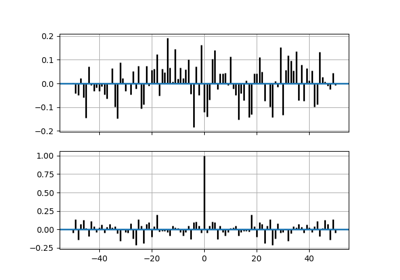You are reading an old version of the documentation (v3.1.3). For the latest version see https://matplotlib.org/stable/api/_as_gen/matplotlib.axes.Axes.acorr.html

# matplotlib.axes.Axes.acorr¶

Axes.acorr(self, x, *, data=None, **kwargs)[source]

Plot the autocorrelation of x.

Parameters: x : array-like detrend : callable, optional, default: mlab.detrend_none x is detrended by the detrend callable. This must be a function x = detrend(x) accepting and returning an numpy.array. Default is no normalization. normed : bool, optional, default: True If True, input vectors are normalised to unit length. usevlines : bool, optional, default: True Determines the plot style. If True, vertical lines are plotted from 0 to the acorr value using Axes.vlines. Additionally, a horizontal line is plotted at y=0 using Axes.axhline. If False, markers are plotted at the acorr values using Axes.plot. maxlags : int, optional, default: 10 Number of lags to show. If None, will return all 2 * len(x) - 1 lags. lags : array (length 2*maxlags+1) The lag vector. c : array (length 2*maxlags+1) The auto correlation vector. line : Artist added to the axes of the correlation: LineCollection if usevlines is True. Line2D if usevlines is False. b : Line2D or None Horizontal line at 0 if usevlines is True None usevlines is False. linestyle : Line2D property, optional The linestyle for plotting the data points. Only used if usevlines is False. marker : str, optional, default: 'o' The marker for plotting the data points. Only used if usevlines is False.

Notes

The cross correlation is performed with numpy.correlate() with mode = "full".

Note

In addition to the above described arguments, this function can take a data keyword argument. If such a data argument is given, the following arguments are replaced by data[<arg>]:

• All arguments with the following names: 'x'.

Objects passed as data must support item access (data[<arg>]) and membership test (<arg> in data).

## Examples using matplotlib.axes.Axes.acorr¶Cross- and Auto-Correlation Demo